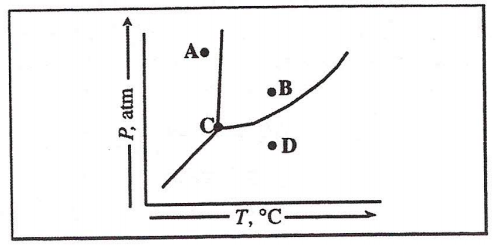# Problem: In the phase diagram, which transition represents the condensation of a gas into a liquid? (A) B to A (B) D to B (C) C to D (D) A to D

###### FREE Expert Solution
84% (492 ratings)
###### Problem Details

In the phase diagram, which transition represents the condensation of a gas into a liquid?

(A) B to A

(B) D to B

(C) C to D

(D) A to DWhat scientific concept do you need to know in order to solve this problem?

Our tutors have indicated that to solve this problem you will need to apply the Phase Diagram concept. You can view video lessons to learn Phase Diagram. Or if you need more Phase Diagram practice, you can also practice Phase Diagram practice problems.

What is the difficulty of this problem?

Our tutors rated the difficulty ofIn the phase diagram, which transition represents the conden...as medium difficulty.

How long does this problem take to solve?

Our expert Chemistry tutor, Sabrina took 1 minute and 39 seconds to solve this problem. You can follow their steps in the video explanation above.

What professor is this problem relevant for?

Based on our data, we think this problem is relevant for Professor Yousif's class at University of Detroit Mercy.